Basic Statistics with Sympathy – Part 4: Building arbitrary RNGs in Sympathy - Combine | Combine

Remember your friend from our very first post? . Well, I am sorry to say that he never really reached French Guyana. He ended up in Carcass, one of the Malvinas/Falkland islands. And his boat was (peacefully) captured by overly friendly pirate penguins. Now he spends his days counting penguins and sheep. He did keep a coin and this calculator as a memento from his past life. You know, just in case.

As for you, you are still at the office and now your task is to get some samples from a distribution that strangely resembles a cropped half co-sine. Your problem is that such distribution is not in NumPy (gasp!). Don’t fret, we got you covered. NumPy not having a distribution should not cause weeping or gnashing of teeth. In fact, your friend can easily build pretty much any distribution with the help of some math, his coin and his trusty office memento (provided that the coin is unbiased and that he has enough patience). This is possible because of the Probability Integral Transform (PIT). As part of this tutorial, we will do the exercise in slide 11 of this lecture, so you might want to open the slides to do the steps together.

One consequence of the PIT is that as long as you can have a random variable in which a cumulative distribution function (CDF) exists, you can then transform the CDF to express the random variable in terms of the uniform distribution. Which means that for a very long list of distributions it would be possible for you to build your own random number generator, using nothing but some simple functions and a uniform distribution sampler!

So, let’s begin with the exercise of the tutorial. We will use our trusty calculator node, so you can place 3 calculator nodes and a dummy input (which can easily be built with a random table generator). We will not use the actual contents of the dummy input, since we will generate our own signals in the calculator. Your start flow may look like this:You can change the labels in the node by double clicking the text. Now, right click on the first calculator node (from left to right) and click “configure”. Make a variable that will be your independent axis through the calculations. If you look in the exercise, the range of the pdf goes from (-pi/2 to pi/2]. Note that the lower bound is an open bound. I will name it x, and you can build it in the calculation field with: np.linspace(-np.pi/2,np.pi/2, num=100)[1:]. You can then double click on the node to run it, in order to have “x” available as an input for the next node, which you will use to build the pdf.

In the calculation field of the node to build the pdf, you simply need to enter the same constraints that appear in the exercise: The pdf is 1/2 cos (x) for x (-pi/2,pi/2].  We could easily deal with that by removing the last sample on every variable.

np.array([0 if x > np.pi/2. or x <= -np.pi/2. else 0.5*np.cos(x) for x in ${x}]) I was first trying out how would the plots look like if I allowed more than the non-zero range to be generated. But you don’t need to do that. You can just do this instead: np.array([0.5*np.cos(x) for x in${x}])

Since we will build a CDF from this PDF, and we will want to plot them together, then is convenient to let the previous signal pass through. So you can simply write a variable named “x”, and drag the variable name in “Signals” of “x” into the calculations field. Your configuration screen will look something like this: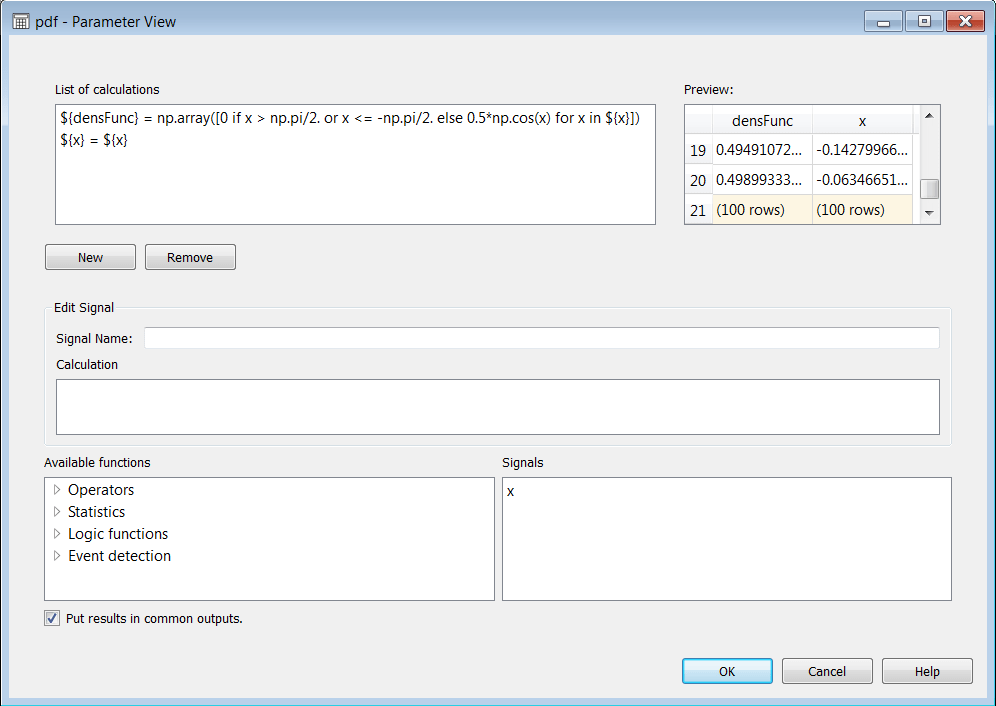We will use densFunc and x to evaluate how well our approximation behaves in the end.

Now, building a CDF from this easily integrable analytic function is quite simple. You can go the analytic or the numeric route. For this tutorial we will also show the numeric route because not all pdfs you may run into will have an easily integrable analytic form. We use the trapezoidal rule just to show that the PIT will approximate the original random variable X even with a not-so-smooth approximation of the CDF. To build the CDF, we take each value of the integration and sum it with the previous value, like so:

np.array( [ ( (${x}[index]-${x}[index-1]) * (${densFunc}[index]+${densFunc}[index-1])/2.) + np.sum([ ( (${x}[indexK]-${x}[indexK-1]) * (${densFunc}[indexK]+${densFunc}[indexK-1])/2.) if indexK>0 else 0 for indexK in range(index) ]) if index>0 else 0 for index in range(0,len(${x})) ]) To analytically build a the CDF, you can start by integrating 1/2 cos(x) dx. Which will give you 1/2 sin(x) + C. To find C, solve the initial condition sin(pi/2) = 1, and that gives you 1/2 sin(x) + 1/2 as the function from which you can generate a CDF. Now, if we plot the PDF and the CDF together (you can use the plot tables node, and choose x as the independent axis), they will look like this: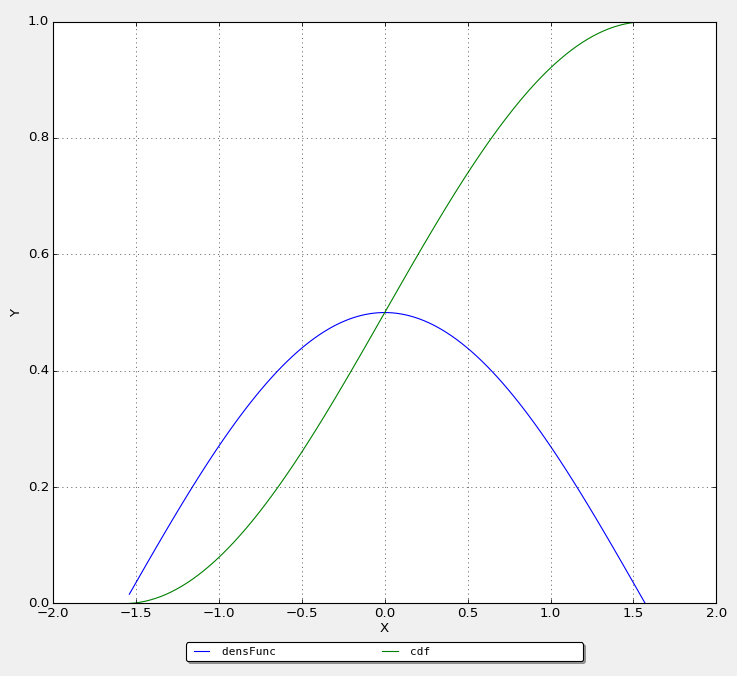Ok, NOW we have all we need to generate some data with the same distribution as X. And we will both use the numeric and the analytic ways. The general way to obtain a function of the uniform distribution is in slide 10 of the presentation, so solving for x: u = 1/2 sin(x) + 1/2 -> x= arcsin(2u-1) You can add a new calculator node, and feed the output of CDF to it. Let us take as many samples as elements exist in our initial independent axis (just for being able to use the same axis across different graphics), and name that simRandVarFromU. You can enter in the calculation field: np.array([np.arcsin(-1+2.*np.random.uniform(0,1)) for b in${x}])

Now, your friend in Carcass Island may actually find it easier to use the numeric way. He probably has a lot of stones and writings on the sand (drawing a lot of slots for his coin to emulate different positions between 0 and 1), and he has been spending a lot of energy scaring away the sheep and the penguins, so let’s make his life a little bit easier. He can calculate numericSim by doing the following:

np.array([${x}[np.where(${cdf}<=np.random.uniform(0,1))[-1]] for inx in ${x}]) See what he did there? he rolled his coin until he got a uniformly distributed number “u” between 0 and 1. Since he knows that the range of the CDF is between 0 and 1, it is safe to see in which value of x was the CDF less than or equal to “u”, and take that as an index to obtain “x”. After building the two estimates of X, let us find a way to compare them. So, what we are ideally constructing is a way of building a variable with a distribution as close as possible to X. So, let’s compare the distribution of: • Original X • PIT generated X (analytical) • PIT generated X (numeric) For both PIT – generated cases, we first obtained a histogram, applied a smoothing over the histogram, and then re-sampled the smooth histogram for a linear space like the one in the independent variable of Original X.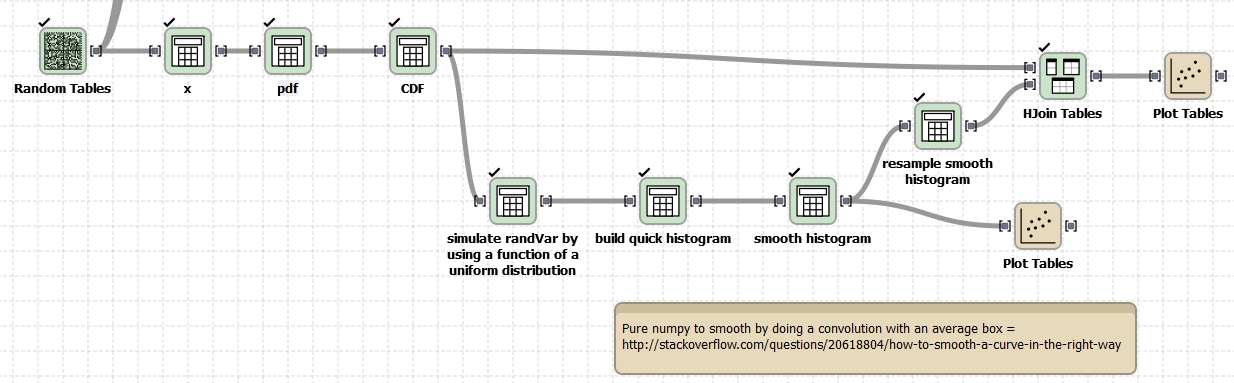To build the histograms: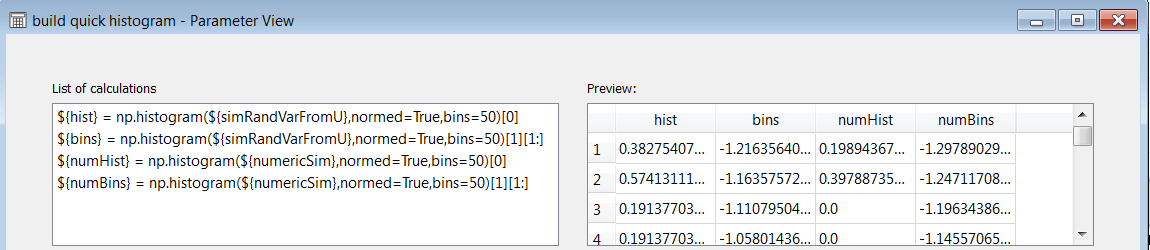Notice that we removed a bin since numpy will generate 1 extra bin. To smooth the histograms, we use a convolution with a window size of 10 samples, like this: np.convolve(${numHist},np.ones(10)/10,mode=’same’)

For both PIT generated histograms. Remember to add all the other signals to be able to make proper plots. So here is a plot of both smooth histograms and their bins: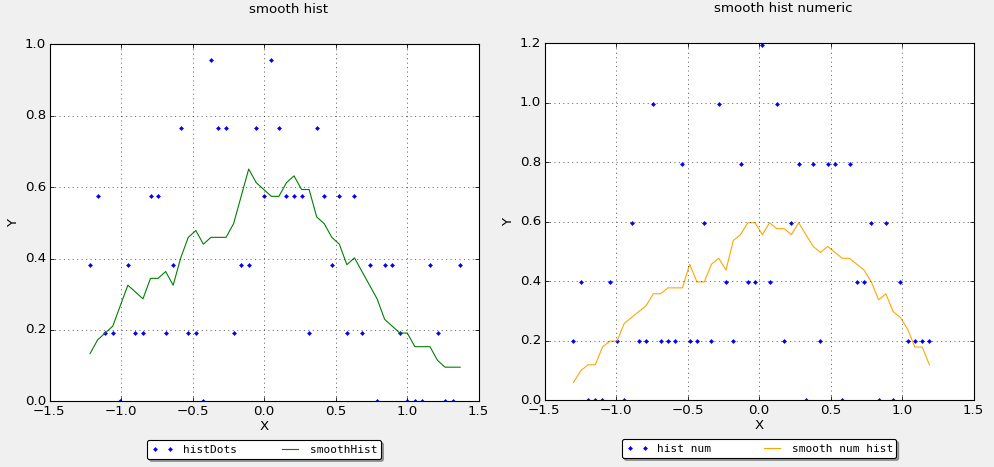To be able to compare the smooth histograms and the real PDF in the same plot, we made an interpolation (to change sample size). We simply did:And after putting together all signals on the same table, we were able to produce this plot: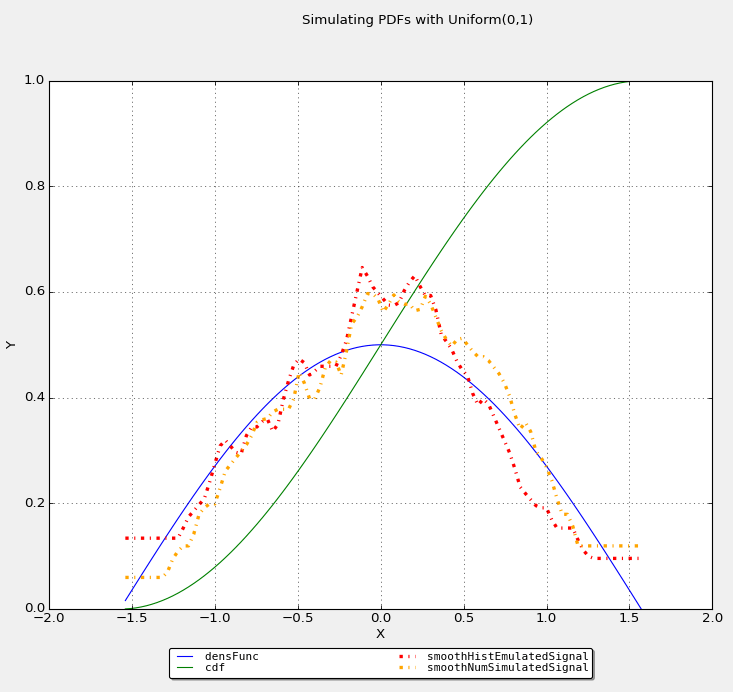So, one can see that at least with 100 samples and a smoothing window of 10 samples, with 50 bins, we got this approximation. What would happen if we start playing with this parameters? First, changing the number of bins at this small amount of samples would not make much difference. Second, getting more samples is very cheap for you in your desktop, but changing a convolution window would be cheaper for your friend in Carcass. An increase of a smoothing window to 23 samples will give us: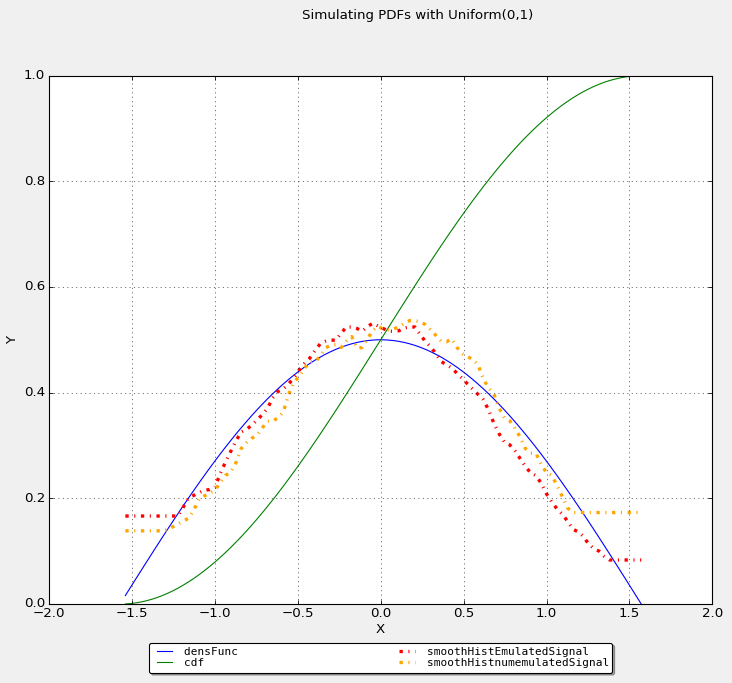But if we really wanted to see if the signals converge together, throw a bigger number of samples. Say, throw 1000 samples, and leave the smoothing window at size 10: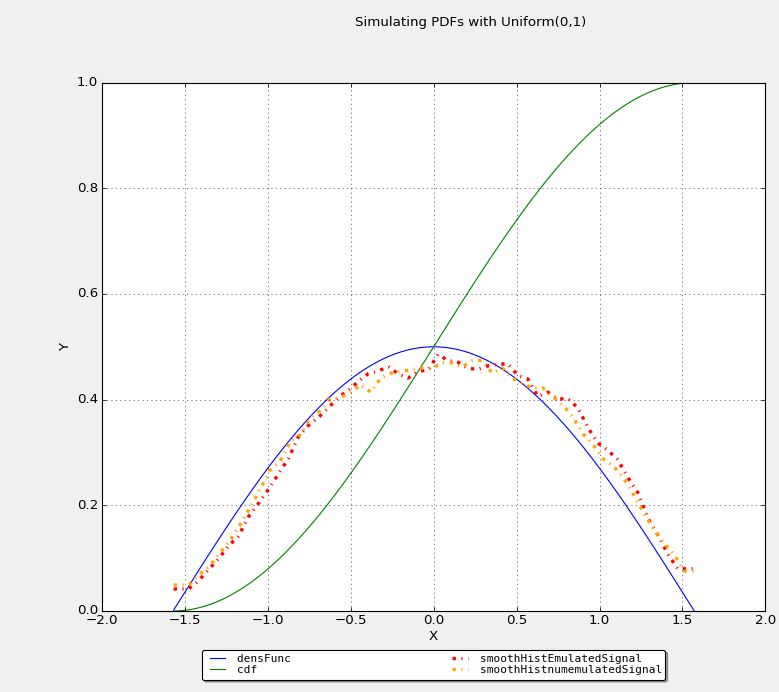I hope that you can add the PIT to your back of tricks, and use it whenever you have the need. Until the next post!

This post borrowed the featured image from here.